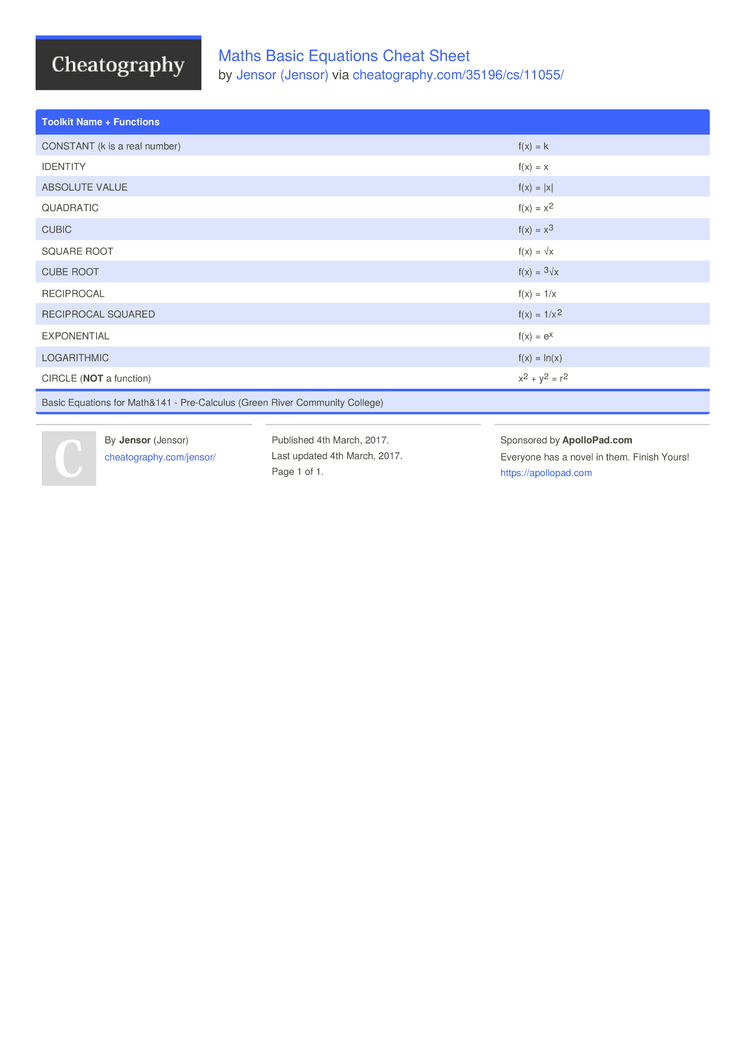# Maths Basic Equations Cheat Sheet by Jensor

Basic toolkit functions

### Toolkit Name + Functions

 CONSTANT (k is a real number) f(x) = k IDENTITY f(x) = x ABSOLUTE VALUE f(x) = |x| QUADRATIC f(x) = x2 CUBIC f(x) = x3 SQUARE ROOT f(x) = √x CUBE ROOT f(x) = 3√x RECIPROCAL f(x) = 1/x RECIPROCAL SQUARED f(x) = 1/x2 EXPONE­NTIAL f(x) = ex LOGARI­THMIC f(x) = ln(x) CIRCLE (NOT a function) x2 + y2 = r2
Basic Equations for Math&141 - Pre-Ca­lculus (Green River Community College)1 Page
//media.cheatography.com/storage/thumb/jensor_maths-basic-equations.750.jpg

PDF (recommended)